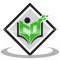## A copper wire, $3 \mathrm{~mm}$ in diameter, is wound about a cylinder whose length is $12 \mathrm{~cm}$, and diameter $10 \mathrm{~cm}$, so as to cover the curved surface of the cylinder. Find the length and mass of the wire, assuming the density of copper to be $8.88 \mathrm{~g} \mathrm{per} \mathrm{cm}^{3}$.Updated on 10-Oct-2022 13:47:38
Given:A copper wire, $3 \mathrm{~mm}$ in diameter, is wound about a cylinder whose length is $12 \mathrm{~cm}$, and diameter $10 \mathrm{~cm}$, so as to cover the curved surface of the cylinder.The density of copper is $8.88 \mathrm{~g} \mathrm{per} \mathrm{cm}^{3}$.To do:We have to find the length and mass of the wire.Solution:Diameter of the cylinder $(d)= 10\ cm$This implies, Radius of the cylinder $(r) = \frac{10}{2}\ cm$$= 5\ cmLength of the wire one complete round = 2 \pi r$$= 2\times3.14\times5\ cm$$= 31.4\ cmThe diameter of the wire = 3\ mm$$= \frac{3}{10}\ cm$This implies, Radius ... Read More

## Formulate the following problems as a pair of equations, and hence find their solutions:(i) Ritu can row downstream 20 km in 2 hours, and upstream 4 km in 2 hours. Find her speed of rowing in still water and the speed of the current.(ii) 2 women and 5 men can together finish an embroidery work in 4 days, while 3 women and 6 men can finish it in 3 days. Find the time taken by 1 woman alone to finish the work, and also that taken by 1 man alone.(iii) Roohi travels 300 km to her home partly by train and partly by bus. She takes 4 hours if she travels 60 km by train and the remaining by bus. If she travels 100 km by train and the remaining by bus, she takes 10 minutes longer. Find the speed of the train and the bus separatelyUpdated on 10-Oct-2022 13:47:38

## Solve the following equations and check your results.$2 y+\frac{5}{3}=\frac{26}{3}-y$.Updated on 10-Oct-2022 13:47:38
Given:$2 y+\frac{5}{3}=\frac{26}{3}-y$To do: We have to solve the given equation and check the result.Solution:$2 y+\frac{5}{3}=\frac{26}{3}-y$$2y+y=\frac{26}{3}-\frac{5}{3}$$3y=\frac{26-5}{3}$$3y=\frac{21}{3}$$3y=7$$y=\frac{7}{3}Substituting the value of y in LHS, we get,2y+\frac{5}{3}=2(\frac{7}{3})+\frac{5}{3}$$=\frac{14}{3}+\frac{5}{3}$$=\frac{14+5}{3}$$=\frac{19}{3}$Substituting the value of $y$ in RHS, we get,$\frac{26}{3}-y=\frac{26}{3}-\frac{7}{3}$$=\frac{26-7}{3}$$=\frac{19}{3}$LHS $=$ RHSThe value of $y$ is $\frac{7}{3}$.

## Solve the following equations and check your results.$\frac{2 x}{3}+1=\frac{7 x}{15}+3$.Updated on 10-Oct-2022 13:47:38
Given:$\frac{2 x}{3}+1=\frac{7 x}{15}+3$To do: We have to solve the given equation and check the result.Solution:$\frac{2 x}{3}+1=\frac{7 x}{15}+3$$\frac{2 x}{3}-\frac{7 x}{15}=3-1$$\frac{5(2 x)-7x}{15}=2$           (LCM of 3 and 15 is 15)$\frac{10x-7x}{15}=2$$\frac{3 x}{15}=2$$3x=15(2)$$3x=30$$x=\frac{30}{3}$$x=10Substituting the value of x in LHS, we get,\frac{2 x}{3}+1=\frac{2 (10)}{3}+1$$=\frac{20}{3}+1$$=\frac{20+1(3)}{3}$$=\frac{20+3}{3}$$=\frac{23}{3}Substituting the value of x in RHS, we get,\frac{7 x}{15}+3=\frac{7 (10)}{15}+3$$=\frac{70}{15}+3$$=\frac{70+3(15)}{15}$$=\frac{70+45}{15}$$=\frac{115}{15}$$=\frac{23}{3}$LHS $=$ RHSThe value of $x$ is $10$.

## Solve the following equations and check your results.$x=\frac{4}{5}(x+10)$.Updated on 10-Oct-2022 13:47:38